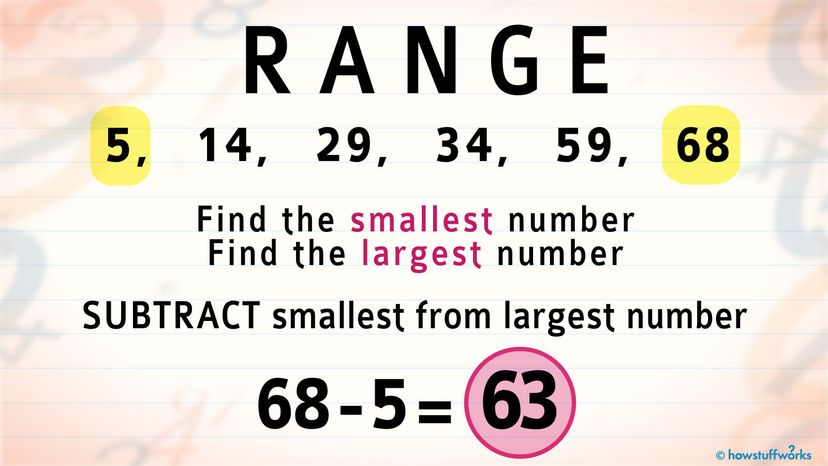# How to Find RangeFinding the range of a series of random numbers is as easy as subtracting the smallest from the largest. HowStuffWorks

The range of a collection of numbers — mathematicians call this a "data set" — is the difference between the highest number and the lowest number in the data set. It tells you how spread out the numbers in the data set are.

Let's say you want to calculate how much you spent on gas on a particular road trip; it's useful to figure out the price you most commonly paid at the tank, as well as the average price and the price in the exact middle. But the range can tell you what the highest and lowest prices were. Range is commonly used by statisticians to figure out the parameters of a data set.

Finding the range is easy. To do it, you just subtract the smallest number in the data set from the largest number. Or, you can use this formula: range = maximum value – minimum value.

Here's a sample data set:

5, 14, 29, 34, 59, 68

In this data set, the only numbers you have to worry about are the lowest (5) and the highest (68). The range of this data set can be found by following the formula:

68-5 = 63

Range can be helpful, but it's limited because it includes all values — even the outliers. For instance, if a data set includes a bunch of clustered numbers and one that's hanging out way out in left field (for instance: 4, 5, 8, 12, 23, 162), calculating the range might not be too helpful.

On the other hand, range can be helpful when you're trying to figure out just how spread out the numbers in a data set actually are.